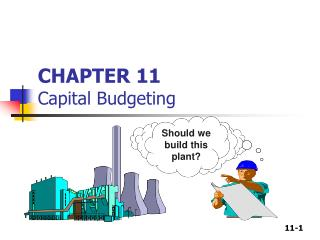DownloadDownload PresentationCHAPTER 11 Capital Budgeting

# CHAPTER 11 Capital Budgeting

Download Presentation## CHAPTER 11 Capital Budgeting

- - - - - - - - - - - - - - - - - - - - - - - - - - - E N D - - - - - - - - - - - - - - - - - - - - - - - - - - -
##### Presentation Transcript

1. CHAPTER 11Capital Budgeting Should we build this plant?

2. What is capital budgeting? • Process of identifying & selecting investments in long-lived assets, (assets that can generate benefits over more than a year). • Analysis of potential additions to fixed assets. • Long-term decisions; involve large expenditures. • Very important to firm’s future.

3. Why do we need capital budgeting? 1) Outlay of capital (initial investment) - immediately. 2) Benefits/return on investment - only after completed / over a no. of years. So?????

4. Steps to capital budgeting • Estimate CFs (inflows & outflows). • Assess riskiness of CFs. • Determine the appropriate cost of capital. • Find NPV and/or IRR. • Accept if NPV > 0 and/or IRR > WACC.

5. What is the difference between independent and mutually exclusive projects? • Independent projects – if the cash flows of one are unaffected by the acceptance of the other. • Mutually exclusive projects – if the cash flows of one can be adversely impacted by the acceptance of the other.

6. Capital budgeting techniques 1) Net Present Value (NPV) 2) Internal Rate of Return (IRR) 3) Modified Internal Rate of Return (MIRR) 4) Payback Period 5) Discount Payback Period

7. 1) Net Present Value (NPV) • Sum of the PVs of all cash inflows and outflows of a project:

8. What is Project L’s NPV? YearCFtPV of CFt 0 -100 ? 1 10 ? 2 60 ? 3 80 ? NPVL = ? If I/YR = 10, find NPVL ?

9. Solving for NPV:Financial calculator solution • Enter CFs into the calculator’s CFLO register. • CF0 = -100 • CF1 = 10 • CF2 = 60 • CF3 = 80 • Enter I/YR = 10, press NPV button to get NPVL = \$18.78.

10. Rationale for the NPV method NPV = PV of inflows – Cost = Net gain in wealth • If projects are independent, accept if the project NPV > 0. • If projects are mutually exclusive, accept projects with the highest positive NPV, (add the most value). • If Project S NPV is \$20.01. • So, accept S if mutually exclusive (NPVs > NPVL) = (\$20.01>\$18.79), & accept both if independent.

11. 2) Internal Rate of Return (IRR) • Indicate what rate of return does a project earn. • IRR is the discount rate that forces PV of inflows equal to cost (IO), and the NPV = 0: • Solving for IRR with a financial calculator: • Press IRR; IRRL = 18.13%

12. Rationale for the IRR method • If IRR > WACC, the project’s return exceeds its costs and there is some return left over to boost stockholders’ returns. If IRR > WACC, accept project. If IRR < WACC, reject project.

13. If 2 projects ( L & S) L’s IRR = 18.13 % S’s IRR = 23.6 % Decision? • If projects are independent, accept both projects, as both IRR > WACC = 10%. • If projects are mutually exclusive, accept S, because IRRs > IRRL.

14. 3) Modified Internal Rate of Return (MIRR) • MIRR is the discount rate that causes the PV of a project’s terminal value (TV) to equal the PV of costs. • TV is found by compounding inflows at WACC. • MIRR assumes cash flows are reinvested at the WACC.

15. 0 1 2 3 10% -100.0 10.0 60.0 80.0 10% 66.0 12.1 10% MIRR = 16.5% 158.1 \$158.1 (1 + MIRRL)3 -100.0 TV inflows \$100 = PV outflows MIRRL = 16.5% Calculating MIRR

16. Why use MIRR versus IRR? • MIRR assumes reinvestment at the opportunity cost = WACC. • Managers like rate of return comparisons, and MIRR is better for this than IRR.

17. 4) Payback period? • The number of years required to recover a project’s cost, or • “How long does it take to get our money back?” • Calculated by adding project’s cash inflows to its cost until the cumulative cash flow for the project turns positive.

18. Calculating payback Project L’s Payback Calculation 3 0 1 2 80 CFt-100 10 60 Cumulative -100 -90 50 -30 30 80 PaybackL = 2 + / = 2.375 years = PaybackL = 2.375 years

19. Strengths and weaknesses of payback • Strengths • Indicate project’s risk and liquidity. • Easy to calculate and understand. • Weaknesses • Ignores the time value of money. • Ignores CFs occurring after the payback period.

20. 5) Discounted payback period • Uses discounted cash flows rather than raw CFs. 3 0 1 2 10% CFt -100 10 60 80 PV of CFt -100 9.09 49.59 60.11 Cumulative -100 -90.91 18.79 -41.32 Disc PaybackL = 2 + / = 2.7 years = 41.32 60.11

21. TQ FOR LISTENING PLZ DO SOME EXERCISES GD LUCK 4 UR FINAL EXAM HAPPY EID MUBARAK & MAAF ZAHIR BATIN